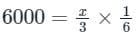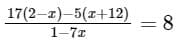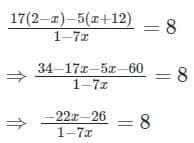Test: Linear Equations in One Variable - 2

# Test: Linear Equations in One Variable - 2 - Class 10

Test Description

## 15 Questions MCQ Test The Complete SAT Course - Test: Linear Equations in One Variable - 2

Test: Linear Equations in One Variable - 2 for Class 10 2023 is part of The Complete SAT Course preparation. The Test: Linear Equations in One Variable - 2 questions and answers have been prepared according to the Class 10 exam syllabus.The Test: Linear Equations in One Variable - 2 MCQs are made for Class 10 2023 Exam. Find important definitions, questions, notes, meanings, examples, exercises, MCQs and online tests for Test: Linear Equations in One Variable - 2 below.
Solutions of Test: Linear Equations in One Variable - 2 questions in English are available as part of our The Complete SAT Course for Class 10 & Test: Linear Equations in One Variable - 2 solutions in Hindi for The Complete SAT Course course. Download more important topics, notes, lectures and mock test series for Class 10 Exam by signing up for free. Attempt Test: Linear Equations in One Variable - 2 | 15 questions in 25 minutes | Mock test for Class 10 preparation | Free important questions MCQ to study The Complete SAT Course for Class 10 Exam | Download free PDF with solutions
 1 Crore+ students have signed up on EduRev. Have you?
Test: Linear Equations in One Variable - 2 - Question 1

### The sum of three consecutive number is 126. Find the highest number?

Detailed Solution for Test: Linear Equations in One Variable - 2 - Question 1

Let the three consecutive number be x, x + 1, x + 2

⇒ x + (x + 1) + (x + 2) = 126

⇒ 3x + 3 = 126

⇒ 3x = 123

⇒ x = 41

∴ Highest number = x + 2 = 41 + 2 = 43

Test: Linear Equations in One Variable - 2 - Question 2

### In a group of 100 students, every student study 8 subjects and every subject is studied by 10 students. The number of subjects is:

Detailed Solution for Test: Linear Equations in One Variable - 2 - Question 2

Total students = 100

According to the question

Every students study 8 subjects, then total subjects = 8 × 100 = 800

If every subject is studied by 10 students, then number of subjects = 800/10 = 80

Test: Linear Equations in One Variable - 2 - Question 3

### Find the 13/16 worth of land whose 9/7 worth be Rs. 10116.

Detailed Solution for Test: Linear Equations in One Variable - 2 - Question 3

Let the total worth of land is Rs. X

⇒ Given, 9x/7 = 10116

⇒ So, x = 7868

∴ 13/16 of 7868 = Rs. 6392.75

Test: Linear Equations in One Variable - 2 - Question 4

A total of 324 notes comprising of Rs. 20 and Rs. 50 denominations make a sum of Rs. 12450. The number of Rs. 20 notes is

Detailed Solution for Test: Linear Equations in One Variable - 2 - Question 4

Given:

Total number of notes of Rs. 20 and Rs. 50 = 324

Total Sum = Rs. 12450

Concept used:

Value of note × Number of total notes = Total amount

Calculation:

Let the number of Rs. 20 notes be x.

No. of Rs. 50 notes = 324 – x

⇒ 20 × x + 50 × (324 – x) = 12450

⇒ 20x + 16200 – 50x = 12450

⇒ -30x = 12450 – 16200

⇒ 30x = 3750

⇒ x = 125

∴ The number of Rs. 20 notes is 125.

Test: Linear Equations in One Variable - 2 - Question 5

If 1/3rd of the first of the three consecutive odd numbers is 2 more than 1/5th of the third number, then the second number is?

Detailed Solution for Test: Linear Equations in One Variable - 2 - Question 5

Given:

1/3rd of the first of the three consecutive odd numbers is 2 more than 1/5th of the third number.

Calculation:

Let, the three consecutive odd numbers be x, x + 2 and x + 4 respectively, then

According to the question,

x × (1/3) = (x + 4) × (1/5) + 2

⇒ x/3 = (x + 4 + 10)/5

⇒ 5x = 3x + 42

⇒ 5x – 3x = 42

⇒ 2x = 42

⇒ x = 21

∴ Second odd number is x + 2 = 21 + 2 = 23

Test: Linear Equations in One Variable - 2 - Question 6

If y2 = y + 7, then what is the value of y3?

Detailed Solution for Test: Linear Equations in One Variable - 2 - Question 6

Given y2 = y + 7

Multiplying LHS and RHS by y

⇒ y3 = y2 + 7y

∴ y3 = y + 7 + 7y = 8y + 7 [∵ y2 = y + 7]

Test: Linear Equations in One Variable - 2 - Question 7

Jane won a lottery and gets 1/3rd of the winning amount and donates Rs. 6000 which is 1/6th  of amount he got, find how much the lottery was worth.

Detailed Solution for Test: Linear Equations in One Variable - 2 - Question 7

Calculation:

Let the lottery worth be Rs. x

Winning amount = x/3

Donated amount =∴ x = Rs. 108000

Test: Linear Equations in One Variable - 2 - Question 8

Rajeev was to earn Rs. 500 and a free holiday for seven weeks’ work. He worked for only 5 weeks and earned Rs. 50 and a free holiday. What was the value of the holiday?

Detailed Solution for Test: Linear Equations in One Variable - 2 - Question 8

Given:

500 + 1 holiday = 7 weeks      ----(1)

50 + 1 holiday = 5 weeks      ----(2)

⇒ From (1) - (2)

450 = 2 weeks

1 week = 225 Rs.

⇒ From (1), 500 + 1 holiday = 7 × 225

1 holiday = 1575 - 500

1 holiday = 1075 Rs.

Test: Linear Equations in One Variable - 2 - Question 9

If 80% of my age 6 years ago is the same as 60% of my age after 10 years. What is the product of digits of my present age?

Detailed Solution for Test: Linear Equations in One Variable - 2 - Question 9

Let the present age be x

⇒ Six years ago my age will be = (x - 6)

⇒ Age after 10 years = (x + 10)

According to the question

⇒ 80% of (x – 6) = 60% of (x + 10)

⇒ 4x – 24 = 3x + 30

⇒ x = 54

∴ Product of digits = 5 × 4 = 20

Test: Linear Equations in One Variable - 2 - Question 10

Find the product of two consecutive numbers where four times the first number is 10 more than thrice the second number.

Detailed Solution for Test: Linear Equations in One Variable - 2 - Question 10

Given:

Four times the first number is 10 more than thrice the second number.

Calculation:

Suppose the numbers are ‘a’ and ‘a + 1’.

According to the question :

4a = 3 × (a + 1) + 10

⇒ a = 13

Hence, the numbers are 13 and 14.

∴ Product = 13 × 14 = 182

Test: Linear Equations in One Variable - 2 - Question 11

Find the value of x if 5x = 2

Detailed Solution for Test: Linear Equations in One Variable - 2 - Question 11

Calculation:

Given that, 5x = 2

Divide the equation by 5

⇒ (5x/5) = (2/5)

⇒ x = 2/5

∴ The value of x is 2/5

Test: Linear Equations in One Variable - 2 - Question 12

Value of x, if 5x - 8 = 7

Detailed Solution for Test: Linear Equations in One Variable - 2 - Question 12

Calculation:

Given that

5x - 8 = 7

⇒ 5x = 7 + 8

⇒ 5x = 15

⇒ x =3

∴ The required value is 3.

Test: Linear Equations in One Variable - 2 - Question 13

A woman had only 50 paisa and 1 rupee coins in her purse. The total number of coins were 120 and the total amount was Rs.100. So the number of 1 rupee coins are_______.

Detailed Solution for Test: Linear Equations in One Variable - 2 - Question 13

Total number of coin = 120

Total amount = Rs 100

Calculation

Let the number of coins of 50 p and Re 1 be x and y

According to question

x + y = 120       ----(1)

x/2 + y = 100      ----(2)

Subtract equation (2)

By solving (1) and (2) from equation (1)

⇒ x - x/2 = 120 - 100

⇒ x/2 = 20

We get x = 40 and y = 80

So number of coins of Rs 1 = 80

∴ The required answer is 80

Test: Linear Equations in One Variable - 2 - Question 14

Find x:Detailed Solution for Test: Linear Equations in One Variable - 2 - Question 14⇒ -22x – 26 = 8 - 56x

⇒ 56x - 22x = 26 + 8

⇒ 34x = 34

∴ x = 1

Test: Linear Equations in One Variable - 2 - Question 15

A is 5 years older than B and 10 years younger than C. If the sum of ages of A, B and C together after 5 years is 80 years. Find the present age of B.

Detailed Solution for Test: Linear Equations in One Variable - 2 - Question 15

Let the age of B be x years

So, the age of A = (x + 5)

And the age of C = (x + 5 +10) = (x + 15)

Now, age of B after 5 years = (x + 5)

Age of A after 5 years = (x + 5) + 5 = (x + 10)

Age of C after 5 years = (x + 15) + 5 = (x + 20)

according to question,

sum of their ages after 5 years = 80

So, (x + 5) + (x + 10) + (x + 20) = 80

⇒ 3x + 35 = 80

⇒ 3x = 45

So, x = 15

∴ present age of B = 15 years

## The Complete SAT Course

445 videos|216 docs|171 tests
Information about Test: Linear Equations in One Variable - 2 Page
In this test you can find the Exam questions for Test: Linear Equations in One Variable - 2 solved & explained in the simplest way possible. Besides giving Questions and answers for Test: Linear Equations in One Variable - 2, EduRev gives you an ample number of Online tests for practice

## The Complete SAT Course

445 videos|216 docs|171 tests